##### Calculus Workbook For Dummies with Online Practice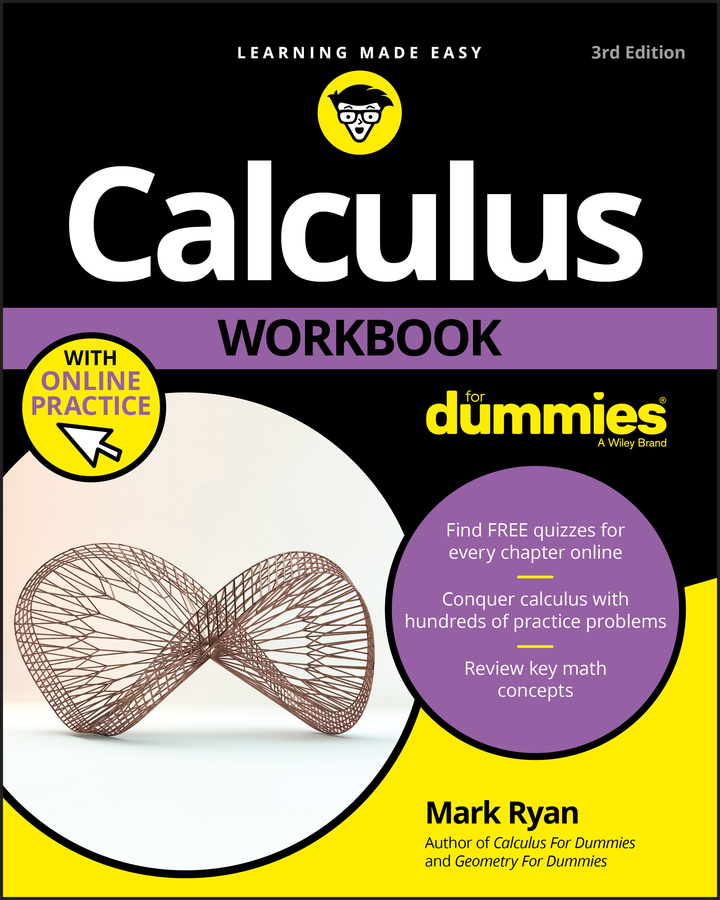You can solve most limit problems by using your calculator. There are two basic methods. For example, say you want to evaluate the following limit: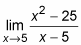## Method one

Here’s what you do. Take a number extremely close to 5 and plug it into x. If you have a Texas Instruments TI-84 calculator, follow these steps:
1. Enter your number, say 4.9999, on the home screen.

2. Press the Sto (store) button, then the x button, and then the Enter button.

This stores the number into x.

3. Enter the function: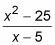4. Hit Enter.

The result, 9.9999, is extremely close to a round number, 10, so that’s your answer.

5. For good measure, store 4.999999 into x.

Follow the procedure in Step 2.

6. Scroll back up to the function by hitting 2nd, Enter, 2nd, Enter.

7. Hit Enter one more time.

You get 9.999999 — even closer to 10. If you still have any doubts, try one more number.

8. Store 4.99999999 into x, scroll up to the function, and Hit Enter.

The result, 10, clinches it. (The function value at 4.99999999 isn’t actually 10, but it’s so close that the calculator rounds it off to 10.)

By the way, if you’re using a different calculator model, you can likely achieve the same result with the same technique or something very close to it.

## Method two

The second calculator method is to produce a table of values:
1. In your calculator’s graphing mode, enter the following: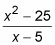2. Go to “table set up” and enter the limit number, 5, as the “table start” number.

3. Enter a small number, say 0.001, for Tbl.

That’s the size of the x-increments in the table.

4. Hit the Table button to produce the table.

5. Scroll up so you can see a couple numbers less than 5.

You should see a table of values like the one in this table.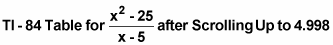X Y
4.998 9.998
4.999 9.999
5 Error
5.001 10.001
5.002 10.002
5.003 10.003
Because y gets very close to 10 as x zeros in on 5 from above and below, 10 is the limit.

These calculator techniques are useful for a number of reasons:

• Your calculator can give you the answers to limit problems that are impossible to do algebraically.

• It can solve limit problems that you could do with paper and pencil except that you’re stumped.

• For problems that you do solve on paper, you can use your calculator to check your answers.

Many calculus problems can be done algebraically, graphically, and numerically. When possible, use two or three of the approaches. Each approach gives you a different take on a problem and enhances your grasp of the relevant concepts.

Use the calculator methods to supplement algebraic methods, but don’t rely too much on them. First of all, the calculator techniques won’t give you an exact answer unless the numbers your calculator gives you are getting close to a number you recognize — like 9.99998 is close to 10, or 0.333332 is close to 1/3.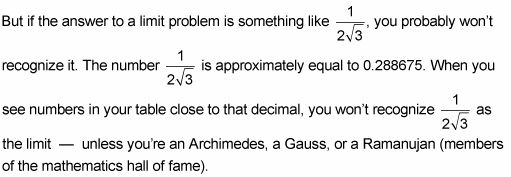However, even when you don’t recognize the exact answer in such cases, you can still learn an approximate answer, in decimal form, to the limit question.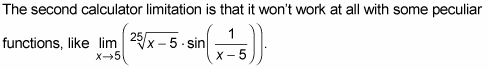This limit equals zero, but you can’t get that result with your calculator.